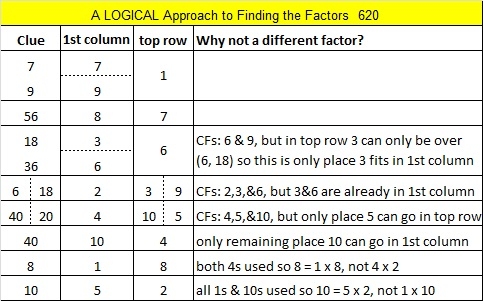# 620 and Level 5

620 is the sum of the four prime numbers from 149 to 163, and it is also the sum of the eight prime numbers from 61 to 97.

620 is the hypotenuse of the Pythagorean triple 372-496-620. What is the greatest common factor of those three numbers?

Today’s puzzle may be a little more difficult than most Level 5 puzzles, but go ahead, embrace the challenge!Print the puzzles or type the solution on this excel file: 10 Factors 2015-09-14

—————————————————————————————————

• 620 is a composite number.
• Prime factorization: 620 = 2 x 2 x 5 x 31, which can be written 620 = (2^2) x 5 x 31
• The exponents in the prime factorization are 2, 1, and 1. Adding one to each and multiplying we get (2 + 1)(1 + 1)(1 + 1) = 3 x 2 x 2 = 12. Therefore 620 has exactly 12 factors.
• Factors of 620: 1, 2, 4, 5, 10, 20, 31, 62, 124, 155, 310, 620
• Factor pairs: 620 = 1 x 620, 2 x 310, 4 x 155, 5 x 124, 10 x 62, or 20 x 31
• Taking the factor pair with the largest square number factor, we get √620 = (√4)(√155) = 2√155 ≈ 24.899799—————————————————————————————————This site uses Akismet to reduce spam. Learn how your comment data is processed.